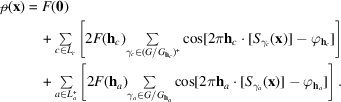International
Tables for
Crystallography
Volume B
Reciprocal space
Edited by U. Shmueli

International Tables for Crystallography (2006). Vol. B, ch. 1.3, p. 69   | 1 | 2 |

Section 1.3.4.2.2.7. Electron-density calculations

G. Bricognea

aMRC Laboratory of Molecular Biology, Hills Road, Cambridge CB2 2QH, England, and LURE, Bâtiment 209D, Université Paris-Sud, 91405 Orsay, France

1.3.4.2.2.7. Electron-density calculations

| top | pdf |

A formula for the Fourier synthesis of electron-density maps from symmetry-unique structure factors is readily obtained by orbit decomposition: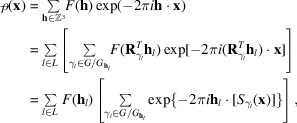where L is a subset ofsuch that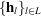contains exactly one point of each orbit for the action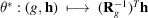of G on. The physical electron density per cubic ångström is then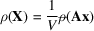with V in Å3.

In the absence of anomalous scatterers in the crystal and of a centre of inversion −I in Γ, the spectrum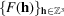has an extra symmetry, namely the Hermitian symmetry expressing Friedel's law (Section 1.3.4.2.1.4). The action of a centre of inversion may be added to that of Γ to obtain further simplification in the above formula: under this extra action, an orbit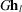with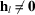is either mapped into itself or into the disjoint orbit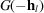; the terms corresponding to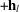and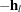may then be grouped within the common orbit in the first case, and between the two orbits in the second case.

 Case 1: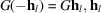is centric. The cosets in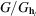may be partitioned into two disjoint classes by picking one coset in each of the two-coset orbits of the action of −I. Let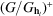denote one such class: then the reduced orbit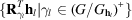contains exactly once the Friedel-unique half of the full orbit, and thus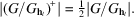Grouping the summands forandyields a real-valued summand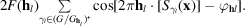Case 2: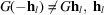is acentric. The two orbits are then disjoint, and the summands corresponding toandmay be grouped together into a single real-valued summand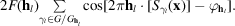In order to reindex the collection of all summands of, put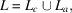where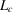labels the Friedel-unique centric reflections in L and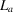the acentric ones, and let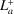stand for a subset ofcontaining a unique element of each pair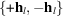for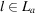. Then